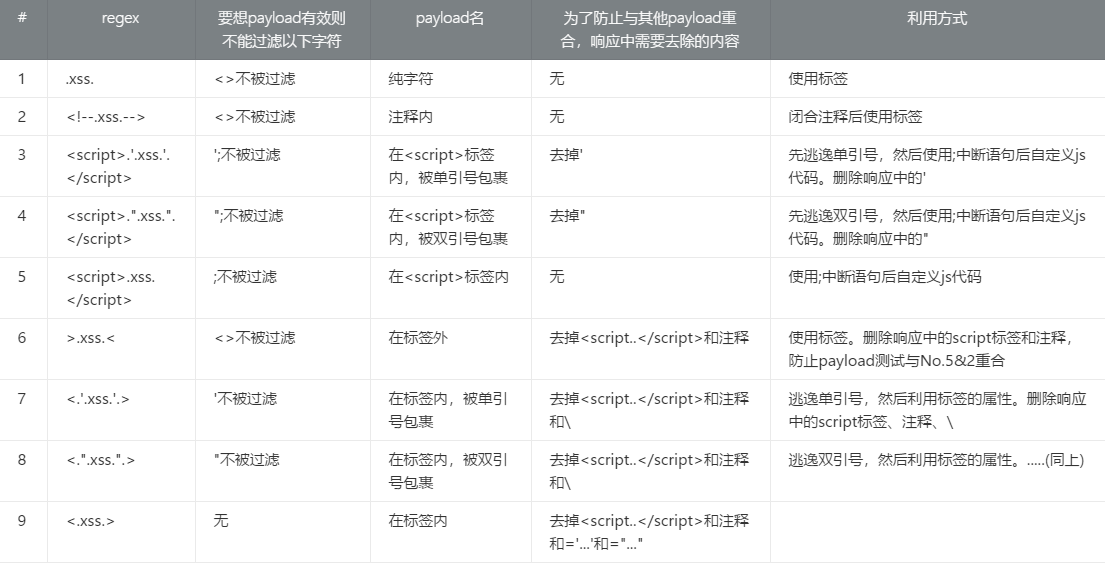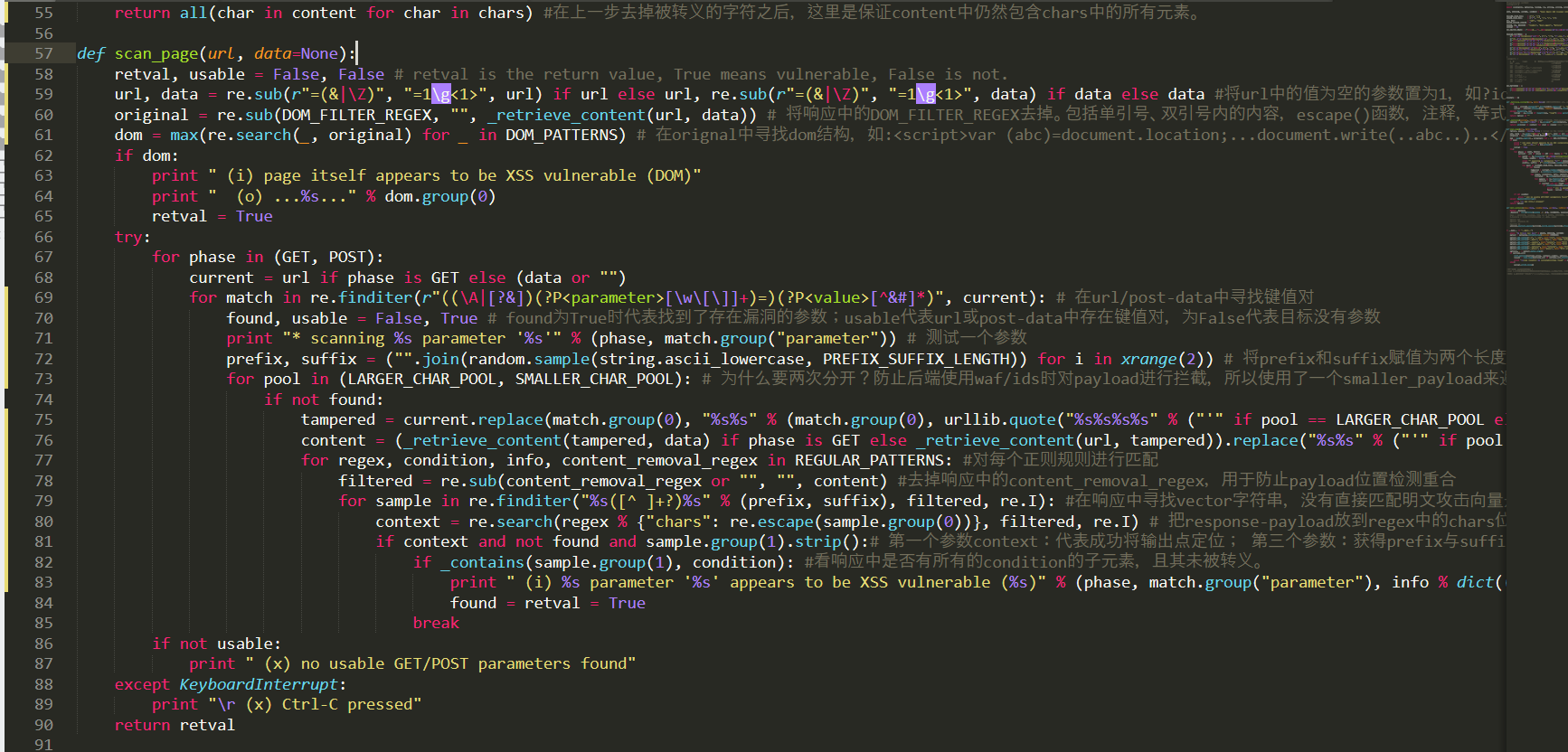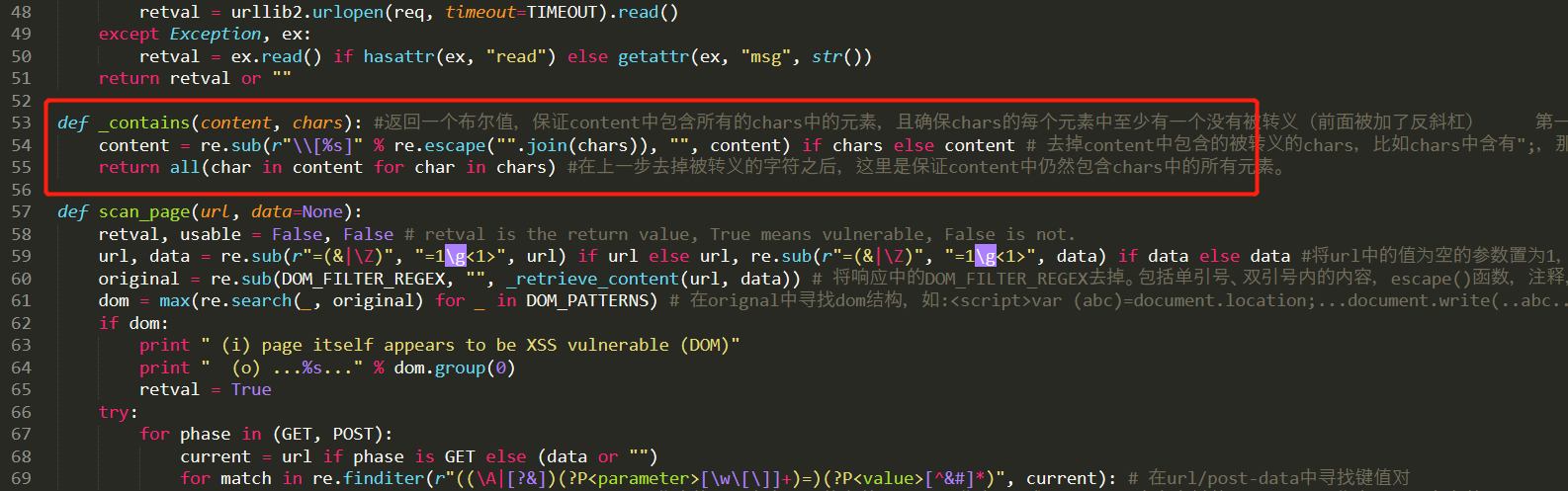# 只有一百行的xss扫描工具——DSXS源码分析

## 0x00 废话

DSXS是一个只有一百行代码的xss扫描器，其作者刚好就是写sqlmap的那位，下面来看一下其扫描逻辑。0x01部分文字较多，感觉写的有点啰嗦，如果看不下去的话就直接看最后的0x02的总结部分。

## 0x01 扫描逻辑

### 第二个逻辑：经过后端的xss

REGULAR_PATTERNS = (                                                                        # each (regular pattern) item consists of (r"context regex", (prerequisite unfiltered characters), "info text", r"content removal regex") # 格式（匹配有可能存在xss的字符串的正则，攻击成功需要哪些字符未经过滤，说明(简易表达匹配格式)，获取响应包后首先筛掉的字符的regexp）
(r"\A[^<>]*%(chars)s[^<>]*\Z", ('<', '>'), "\".xss.\", pure text response, %(filtering)s filtering", None),
(r"<!--[^>]*%(chars)s|%(chars)s[^<]*-->", ('<', '>'), "\"<!--.'.xss.'.-->\", inside the comment, %(filtering)s filtering", None),
(r"(?s)<script[^>]*>[^<]*?'[^<']*%(chars)s|%(chars)s[^<']*'[^<]*</script>", ('\'', ';'), "\"<script>.'.xss.'.</script>\", enclosed by <script> tags, inside single-quotes, %(filtering)s filtering", r"\\'"),
(r'(?s)<script[^>]*>[^<]*?"[^<"]*%(chars)s|%(chars)s[^<"]*"[^<]*</script>', ('"', ';'), "'<script>.\".xss.\".</script>', enclosed by <script> tags, inside double-quotes, %(filtering)s filtering", r'\\"'),
(r"(?s)<script[^>]*>[^<]*?%(chars)s|%(chars)s[^<]*</script>", (';',), "\"<script>.xss.</script>\", enclosed by <script> tags, %(filtering)s filtering", None),
(r">[^<]*%(chars)s[^<]*(<|\Z)", ('<', '>'), "\">.xss.<\", outside of tags, %(filtering)s filtering", r"(?s)<script.+?</script>|<!--.*?-->"),
(r"<[^>]*=\s*'[^>']*%(chars)s[^>']*'[^>]*>", ('\'',), "\"<.'.xss.'.>\", inside the tag, inside single-quotes, %(filtering)s filtering", r"(?s)<script.+?</script>|<!--.*?-->|\\"),
(r'<[^>]*=\s*"[^>"]*%(chars)s[^>"]*"[^>]*>', ('"',), "'<.\".xss.\".>', inside the tag, inside double-quotes, %(filtering)s filtering", r"(?s)<script.+?</script>|<!--.*?-->|\\"),
(r"<[^>]*%(chars)s[^>]*>", (), "\"<.xss.>\", inside the tag, outside of quotes, %(filtering)s filtering", r"(?s)<script.+?</script>|<!--.*?-->|=\s*'[^']*'|=\s*\"[^\"]*\""),
)62-65行是dom-xss检测，是属于上面的第一个逻辑，这里就不再展开。从67行往下看，首先是分别进行GET和POST请求，然后到了69行。

1) .试图触发xss
2) .故意构造一个错误的sql语句用于报错，试图在报错信息中寻找触发点